Want to share your content on R-bloggers? click here if you have a blog, or here if you don't.

What causes the salary to change during the working life of engineers in Sweden?

I will examine data from Statistics Sweden to see what information you can interpret from it.

Statistics Sweden is the Swedish government agency responsible for producing official statistics regarding Sweden, https://www.scb.se/en/.

“Everybody should have the opportunity to find facts and break the myths with our statistics!” Statistics Sweden twitter account, sverigeisiffror.

Salaries are reported for every occupational group SSYK (Standard for Swedish occupational classification). I will examine SSYK 214, architects, engineers and related professionals.

First, define libraries and functions.

```library (tidyverse)
## -- Attaching packages -------------------------------------------- tidyverse 1.2.1 --
## v ggplot2 3.2.0     v purrr   0.3.2
## v tibble  2.1.3     v dplyr   0.8.3
## v tidyr   0.8.3     v stringr 1.4.0
## v readr   1.3.1     v forcats 0.4.0
## -- Conflicts ----------------------------------------------- tidyverse_conflicts() --
library (broom)
library (car)
##
## Attaching package: 'car'
## The following object is masked from 'package:dplyr':
##
##     recode
## The following object is masked from 'package:purrr':
##
##     some
library (splines)
#install_github("ZheyuanLi/SplinesUtils")
library(SplinesUtils)

read_csv (file1, col_types = cols(), locale = readr::locale (encoding = "latin1"), na = c("..", "NA")) %>%
gather (starts_with("19"), starts_with("20"), key = "year", value = salary) %>%
drop_na() %>%
mutate (year_n = parse_number (year))
}```

The data table is downloaded from Statistics Sweden. It is saved as a comma-delimited file without heading, 00000031.csv, http://www.statistikdatabasen.scb.se/pxweb/en/ssd/.

The table: Average monthly pay (total pay), non-manual workers private sector (SLP), SEK by occupational group (SSYK), age, sex and year. Year 2014 – 2018

The average age within each age group is used as a numeric value for graphical presentation and the linear model.

As the salary increases exponentially each year, I have used the logarithm for years in my model.

As a proxy for experience years since graduation can be used. I will simplify by using age as a proxy for experience. I will use a B-spline function to approximate the increase in salaries over age. Spline function is used instead of a polynomial to avoid oscillation.

We expect that both years and age are important factors on the salary. As a null hypothesis, we assume that years and age is not related to the salary and examine if we can reject this hypothesis with the data from Statistics Sweden.

Every year the annual salary negotiation will increase the salaries. As age increases, we acquire more skill at work.

```tb <- readfile("00000031.csv") %>%
rowwise() %>%
mutate(age_l = unlist(lapply(strsplit(substr(age, 1, 5), "-"), strtoi))) %>%
rowwise() %>%
mutate(age_h = unlist(lapply(strsplit(substr(age, 1, 5), "-"), strtoi))) %>%
mutate(age_n = (age_l + age_h) / 2)

tb %>%
ggplot () +
geom_point (mapping = aes(x = year_n,y = salary, colour = age, shape=sex)) +
labs(
x = "Year",
y = "Salary (SEK/month)"
)```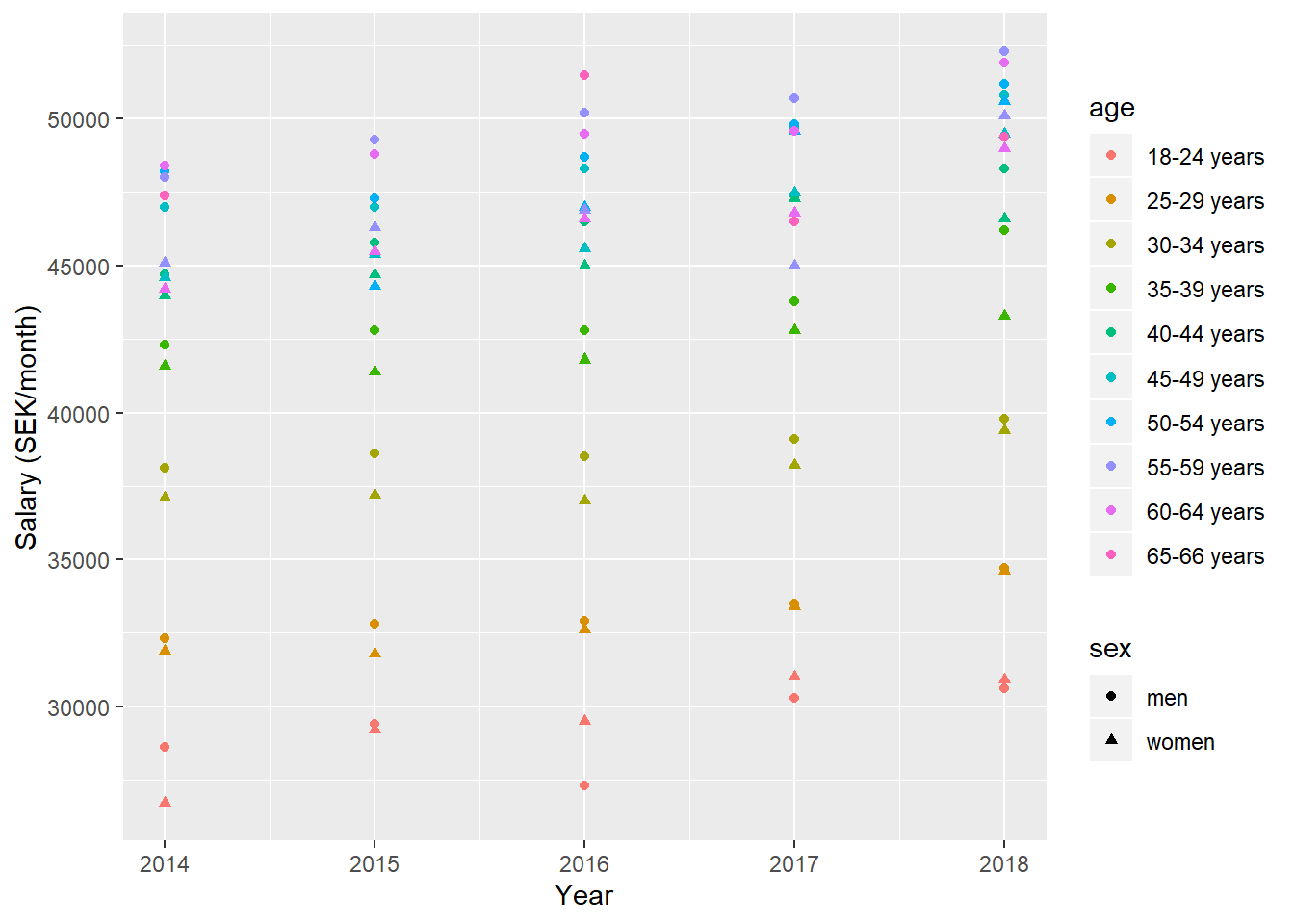Figure 1: SSYK 214, Architects, engineers and related professionals, Year 2014 – 2018

```
model <- lm (log(salary) ~ year_n + sex + bs(age_n, knots = c(30, 40, 50, 60)), data = tb)

tb <- bind_cols(tb, as_tibble(exp(predict(model, tb, interval = "confidence"))))```

From the summary table of the linear model, we can see that the wages have increased by 1,91 % +/- 0,35 % per year with 95 % confidence, holding age as constant. The R-value from the Anova table for years is 120, sufficient for rejecting the null hypothesis that years has no effect on the salary. Holding year as constant, the p values for age are small (< 2e-16) and the R-value from the Anova table value for age is 703, sufficient for rejecting the null hypothesis that experience has no effect on the salary. The adjusted R-squared value is 0,984 implying a good fit of the model.

```tb %>%
ggplot () +
geom_point (mapping = aes(x = year_n,y = fit, colour = age, shape=sex)) +
labs(
x = "Year",
y = "Salary (SEK/month)"
)```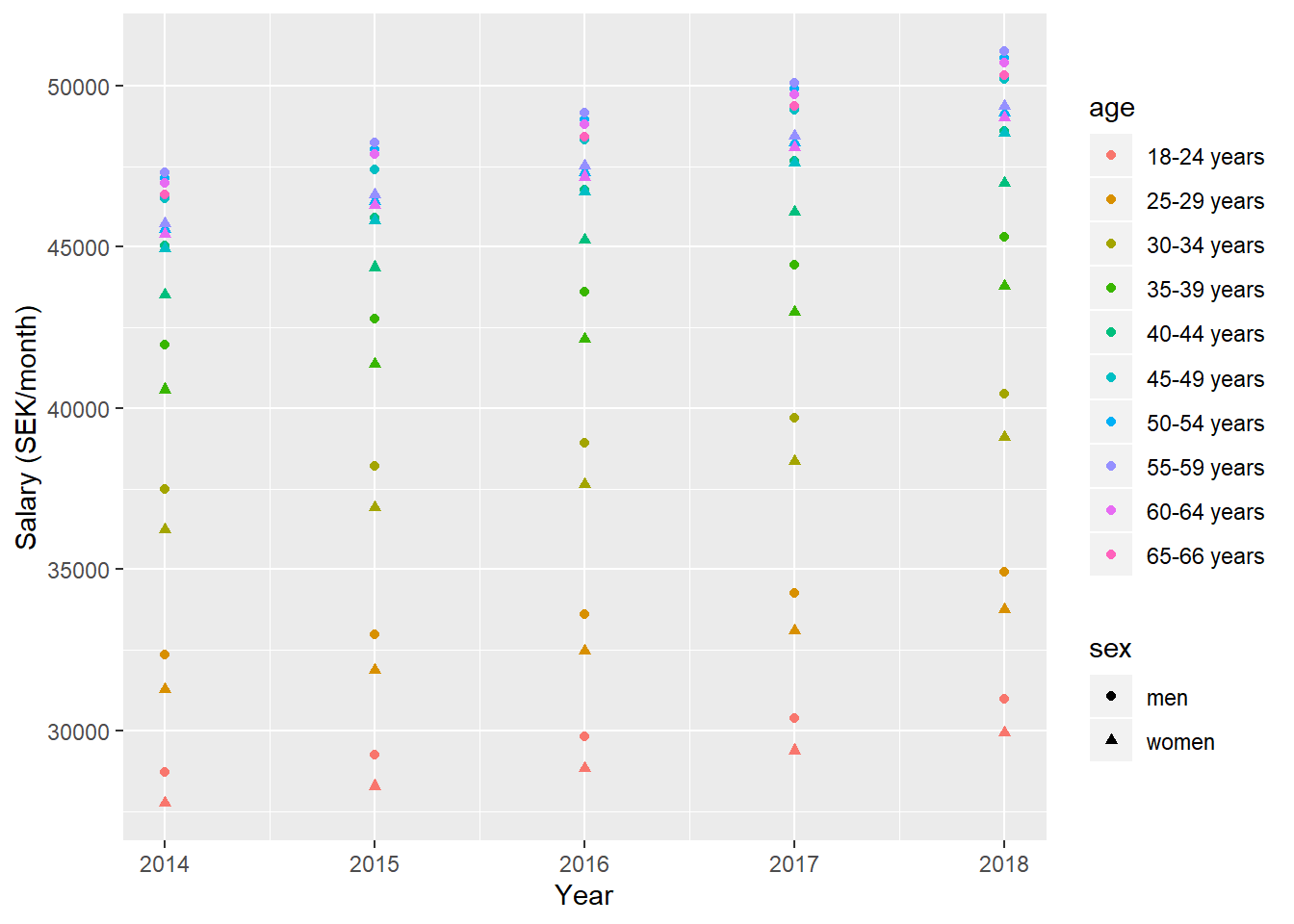Figure 2: Model fit, SSYK 214, Architects, engineers and related professionals, Year 2014 - 2018

```
summary(model) %>%
tidy() %>%
knitr::kable(
booktabs = TRUE,
caption = 'Summary from linear model fit')```
Table 1: Summary from linear model fit
term estimate std.error statistic p.value
(Intercept) -28.1818464 3.5078741 -8.0338820 0.0000000
year_n 0.0190896 0.0017400 10.9709680 0.0000000
sexwomen -0.0338703 0.0050397 -6.7207107 0.0000000
bs(age_n, knots = c(30, 40, 50, 60))1 0.0136922 0.0224921 0.6087566 0.5443286
bs(age_n, knots = c(30, 40, 50, 60))2 0.2417694 0.0207156 11.6708804 0.0000000
bs(age_n, knots = c(30, 40, 50, 60))3 0.4589440 0.0172130 26.6625840 0.0000000
bs(age_n, knots = c(30, 40, 50, 60))4 0.4963401 0.0176805 28.0727805 0.0000000
bs(age_n, knots = c(30, 40, 50, 60))5 0.5045782 0.0195688 25.7848966 0.0000000
bs(age_n, knots = c(30, 40, 50, 60))6 0.4878569 0.0169888 28.7164274 0.0000000
bs(age_n, knots = c(30, 40, 50, 60))7 0.4850548 0.0143718 33.7504146 0.0000000
```summary(model)\$r.squared
##  0.9839054

Anova(model, type=2) %>%
tidy() %>%
knitr::kable(
booktabs = TRUE,
caption = 'Anova report from linear model fit')```
Table 1: Anova report from linear model fit
term sumsq df statistic p.value
year_n 0.0687831 1 120.36214 0
sex 0.0258120 1 45.16795 0
bs(age_n, knots = c(30, 40, 50, 60)) 2.8136569 7 703.36608 0
Residuals 0.0480033 84 NA NA

Let’s have a look at the spline function from the regression model. I use the package SplineUtils to extract the polynomial train from the model coefficients.

```myspline <- RegBsplineAsPiecePoly(model, "bs(age_n, knots = c(30, 40, 50, 60))")

tibble(age_n = 21:65) %>%
ggplot () +
geom_point (mapping = aes(x = age_n,y = predict(myspline, age_n))) +
labs(
x = "Age",
y = "Salary"
)```Figure 3: Model fit, SSYK 214, Correlation between age and salary

The derivative of the spline function for age is equal to the annual salary raise that comes from experience. I also add the salary raise that comes from the annual salary negotiation resulting in the total salary increase distributed over age.

```summod <- tidy(summary (model))

myfun <- function(x){
return (predict(myspline, x, deriv = 1) + summod\$estimate)
}

tibble(age_n = 21:65) %>%
ggplot () +
geom_point (mapping = aes(x = age_n,y = myfun(age_n))) +
labs(
x = "Age",
y = "Salary raise (%)"
)```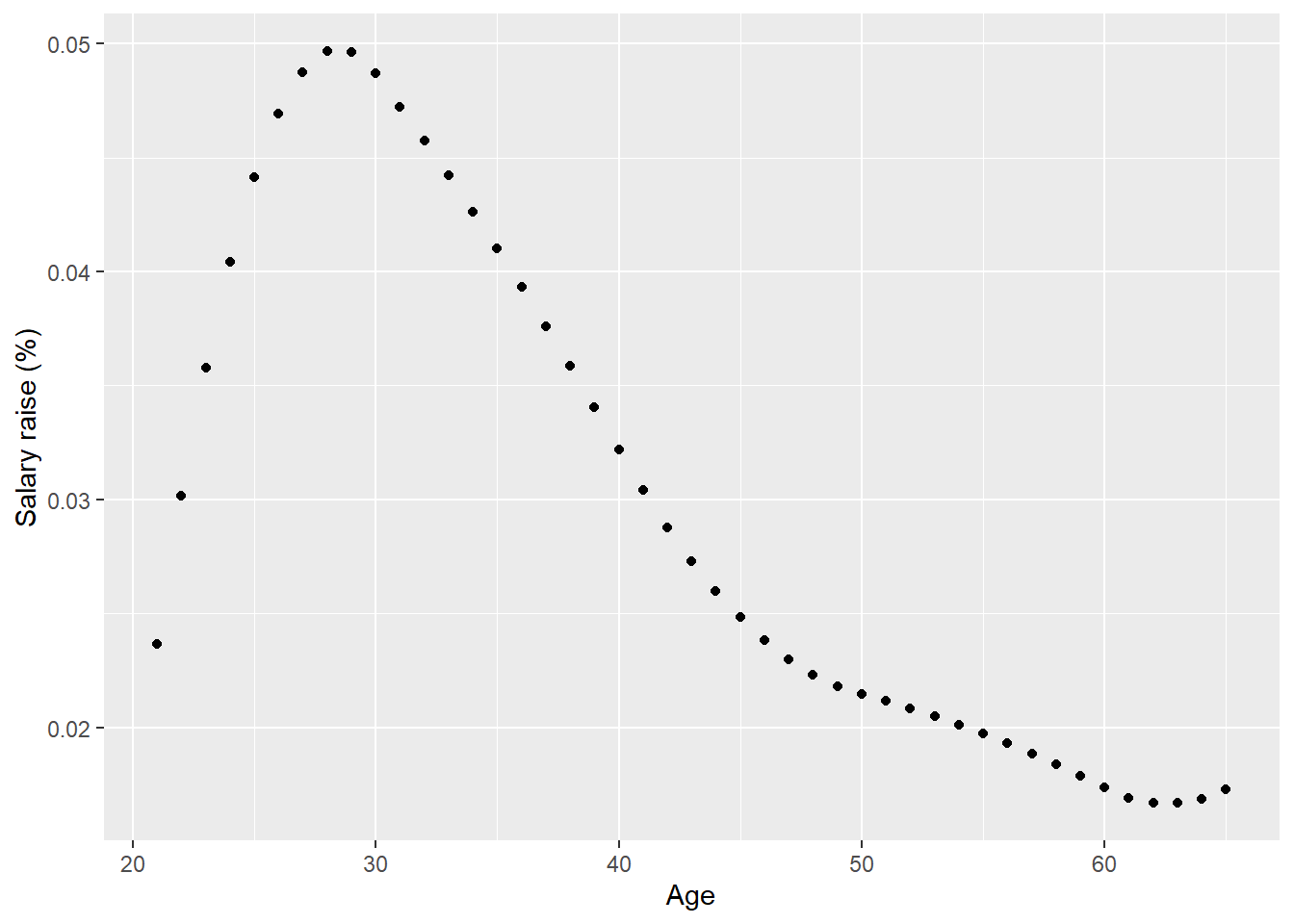Figure 4: Model fit, SSYK 214, The derivative for age

Now. let´s perform some diagnostics on the model. First, a look at the residuals for the model shows no apparent problem.

`plot(model, which = 1)`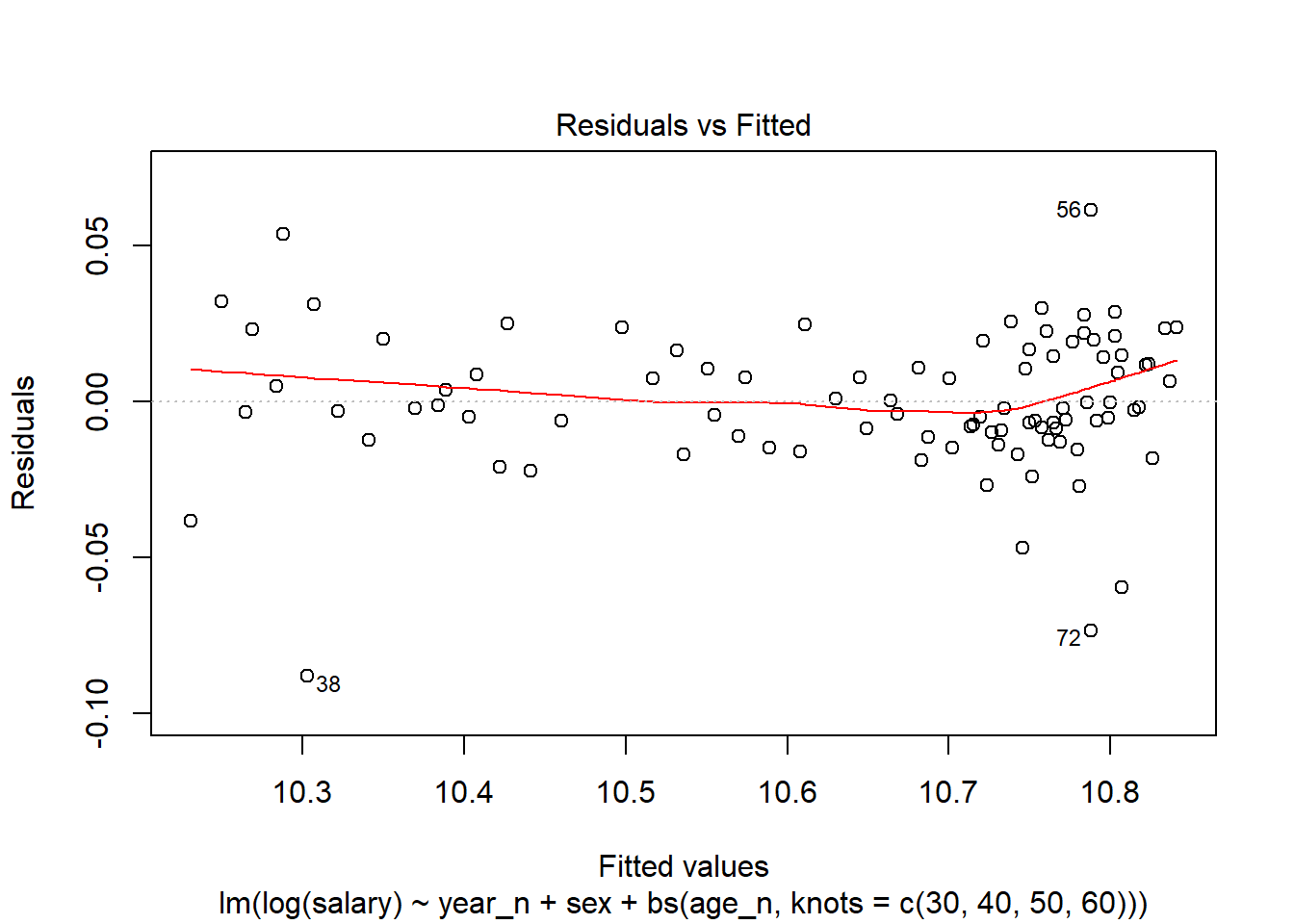Figure 5: Residuals vs Fitted of model fit

The Normal Q-Q shows some possible outliers.

`plot(model, which = 2)`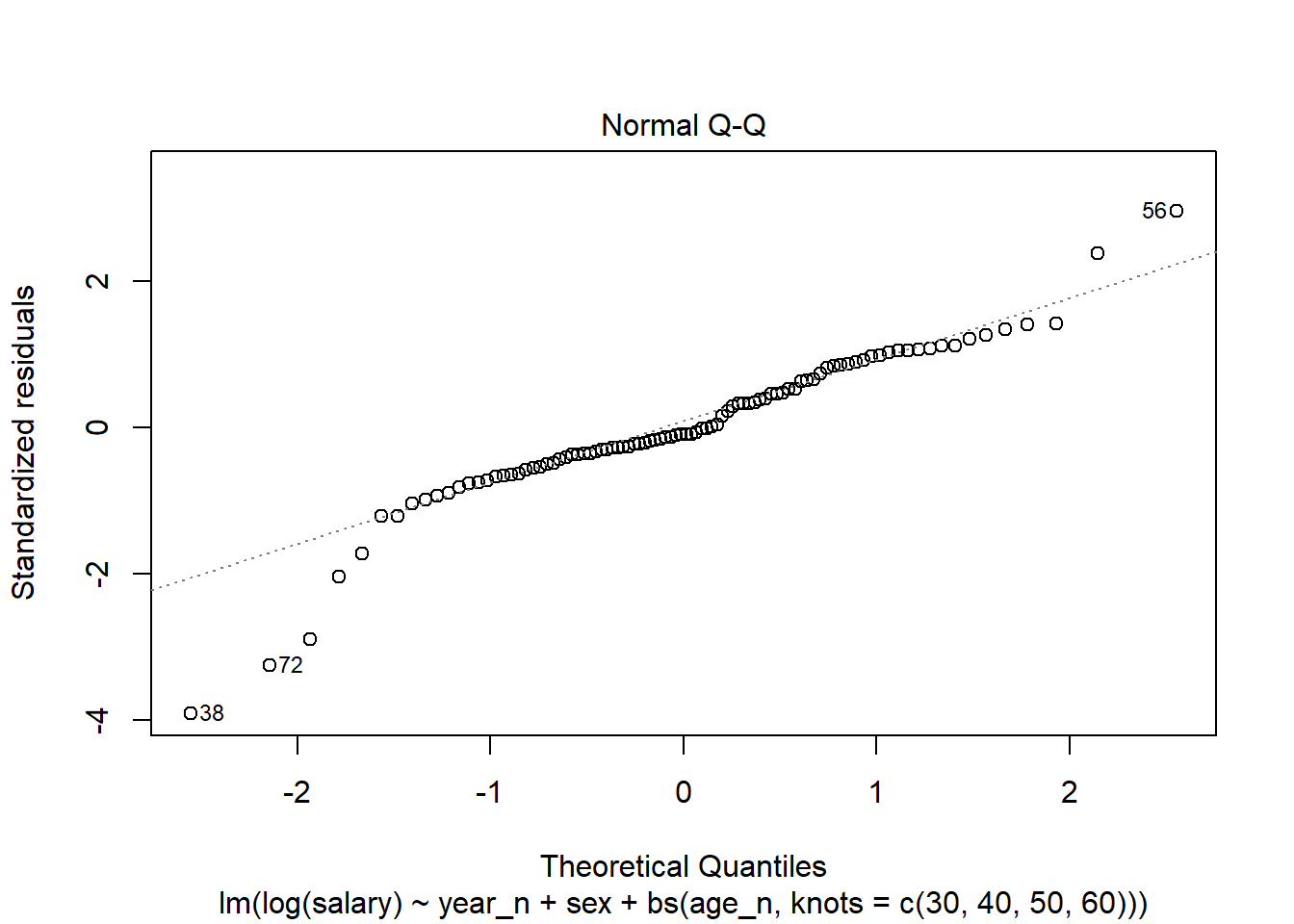Figure 6: Normal Q-Q

Again, the Standardised residuals show some possible outliers.

`plot(model, which = 3)`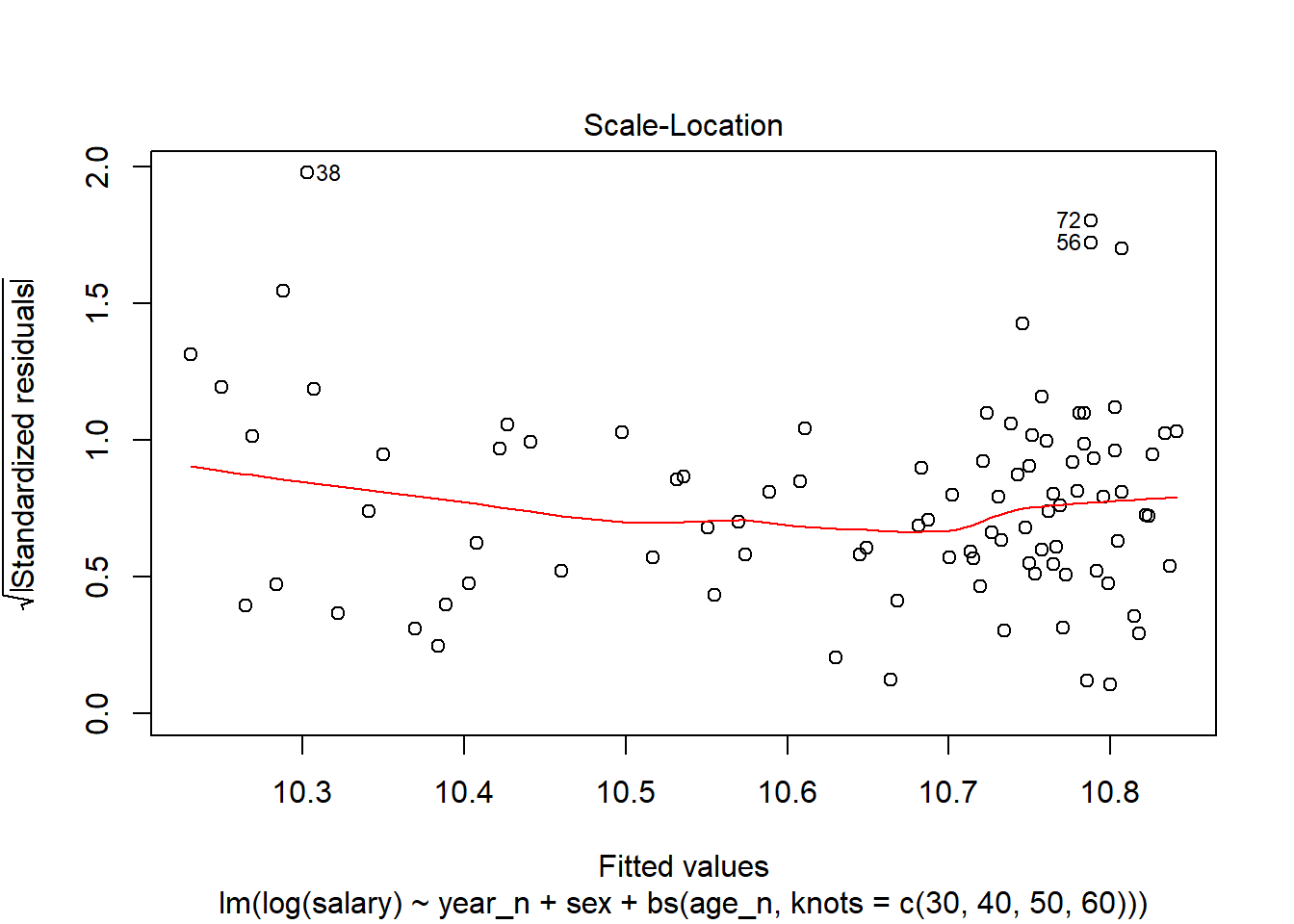Figure 7: Scale-Location

The outliers are also found in the Leverage plot.

`plot(model, which = 5)`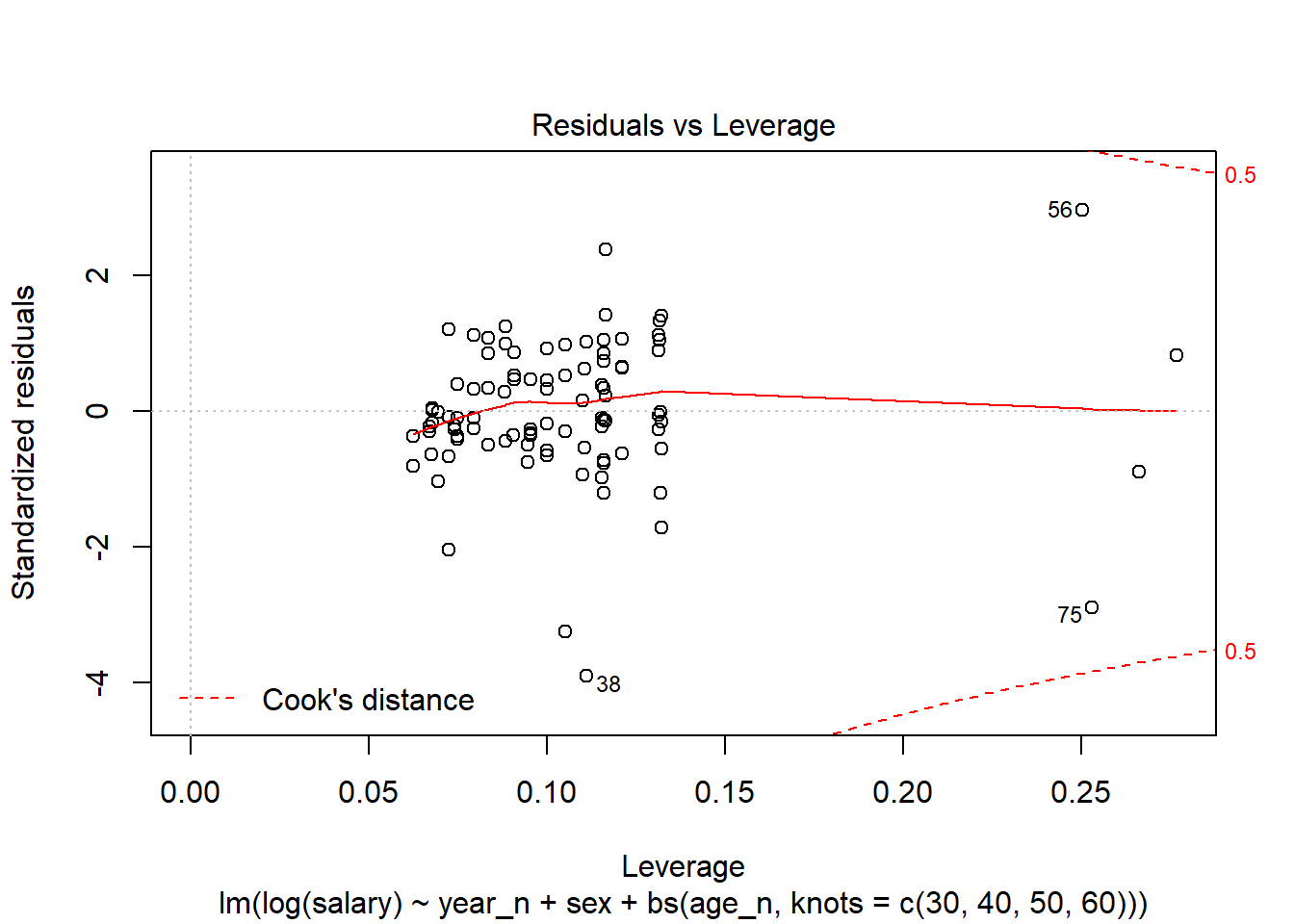Figure 8: Residuals vs Leverage

The outliers 38, 56 and 75 are small groups with larger variance at either end of the age spectrum. Record 72 are women age 55-59.

```tb[38,]\$age
##  "18-24 years"
tb[56,]\$age
##  "65-66 years"
tb[75,]\$age
##  "65-66 years"

tb[72,]
## Source: local data frame [1 x 12]
## Groups:
##
## # A tibble: 1 x 12
##   `occuptional  (~ age   sex   year  salary year_n age_l age_h age_n    fit
##
## 1 214 Engineering~ 55-5~ women 2017   45000   2017    55    59    57 48433.
## # ... with 2 more variables: lwr , upr ```

Wage statistics

The Mediation Institute is responsible for Sweden’s official salary statistics. This applies to three types of statistics: cyclical wage statistics, wage structure statistics and statistics supplied to the European statistical body Eurostat, https://www.mi.se/other-languages/about-the-mediation-office-the-swedish-model-and-wage-statistics-in-english/.

The statistics are collected and processed by Statistics Sweden. It is reported both on Statistics Sweden’s website and the Mediation Institute’s website.

https://www.r-bloggers.com/

https://rweekly.org

Last edited: 2019-12-10